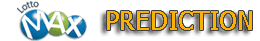# LottoMax Prediction

##Beta 2.16.2022

The theory of this prediction is based on following facts: The number of next draw can be get from the pool of the past draws, not all, but 6 or 8 draws.

So that 5 to 6 numbers of the next draw will be from the about 30 number pool. The hit ratio is increased.

Analysis draws, until 5845draw, offset 0~20draws,
past draws. Compare with the target draw.

 Analysis 14 draws, until 5845. Offset 0 draws, past 6 draws 61÷396=0.1540404040404 Go Analysis 14 draws, until 5845. Offset 1 draws, past 6 draws 59÷395=0.14936708860759 Go Analysis 14 draws, until 5845. Offset 2 draws, past 6 draws 56÷399=0.14035087719298 Go Analysis 14 draws, until 5845. Offset 3 draws, past 6 draws 57÷399=0.14285714285714 Go Analysis 14 draws, until 5845. Offset 4 draws, past 6 draws 52÷403=0.12903225806452 Go Analysis 14 draws, until 5845. Offset 5 draws, past 6 draws 54÷405=0.13333333333333 Go Analysis 14 draws, until 5845. Offset 6 draws, past 6 draws 59÷405=0.14567901234568 Go Analysis 14 draws, until 5845. Offset 7 draws, past 6 draws 62÷407=0.15233415233415 Go Analysis 14 draws, until 5845. Offset 8 draws, past 6 draws 65÷407=0.15970515970516 Go Analysis 14 draws, until 5845. Offset 9 draws, past 6 draws 64÷406=0.1576354679803 Go Analysis 14 draws, until 5845. Offset 10 draws, past 6 draws 61÷403=0.15136476426799 Go Analysis 14 draws, until 5845. Offset 11 draws, past 6 draws 63÷397=0.15869017632242 Go Analysis 14 draws, until 5845. Offset 12 draws, past 6 draws 58÷390=0.14871794871795 Go Analysis 14 draws, until 5845. Offset 13 draws, past 6 draws 56÷385=0.14545454545455 Go Analysis 14 draws, until 5845. Offset 14 draws, past 6 draws 56÷382=0.14659685863874 Go Analysis 14 draws, until 5845. Offset 15 draws, past 6 draws 51÷381=0.13385826771654 Go Analysis 14 draws, until 5845. Offset 16 draws, past 6 draws 47÷378=0.12433862433862 Go Analysis 14 draws, until 5845. Offset 17 draws, past 6 draws 44÷378=0.11640211640212 Go Analysis 14 draws, until 5845. Offset 18 draws, past 6 draws 49÷376=0.13031914893617 Go Analysis 14 draws, until 5845. Offset 19 draws, past 6 draws 53÷377=0.14058355437666 Go Analysis 14 draws, until 5845. Offset 20 draws, past 6 draws 57÷379=0.15039577836412 Go